# Booth lemniscate

A plane algebraic curve of order four whose equation in orthogonal Cartesian coordinates is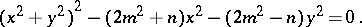If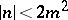, the Booth lemniscate is called elliptic (it has singular point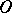(Fig. a), where). If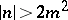, the Booth lemniscate is called hyperbolic (it has a nodal point at the coordinate origin, cf. Fig. b, where).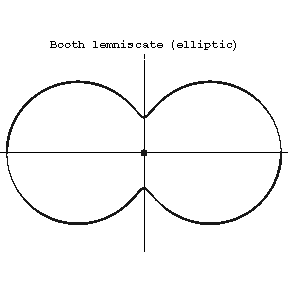Figure: b017000aFigure: b017000b

The equation of an elliptic Booth lemniscate in polar coordinates is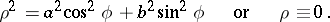If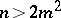, the equation of a hyperbolic Booth lemniscate has the form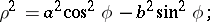and if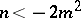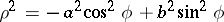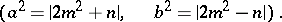The arc length of a Booth lemniscate is expressed by elliptic integrals. The area bounded by an elliptic Booth lemniscate is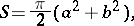while that bounded by a hyperbolic Booth lemniscate is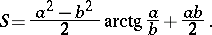The Booth lemniscate is a special case of a Persian curve.

Named after J. Booth .